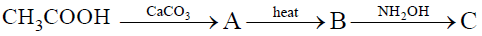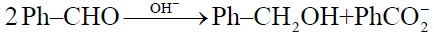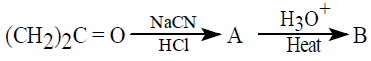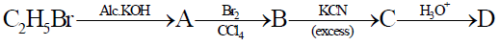Courses

# Test: Chemistry- 5

## 45 Questions MCQ Test NEET Mock Test Series | Test: Chemistry- 5

Description
This mock test of Test: Chemistry- 5 for NEET helps you for every NEET entrance exam. This contains 45 Multiple Choice Questions for NEET Test: Chemistry- 5 (mcq) to study with solutions a complete question bank. The solved questions answers in this Test: Chemistry- 5 quiz give you a good mix of easy questions and tough questions. NEET students definitely take this Test: Chemistry- 5 exercise for a better result in the exam. You can find other Test: Chemistry- 5 extra questions, long questions & short questions for NEET on EduRev as well by searching above.
QUESTION: 1

### Crotonaldehyde (CH3 CH = CHCHO)can be easily oxidized to crotonic acid (CH3 CH = CHCOOH) using

Solution:

KMnO4 oxidise double bond to  form diols whereas Triple bond to form Carboxylic acid. Thus tollen reagent is used for the conversion.

QUESTION: 2

Solution:
QUESTION: 3

### When propyne is treated with aqueous H2SO4 in presence of HgSO4 the major product obtained is

Solution:
QUESTION: 4
Which of the following compounds is oxidised to prepare ethyl methyl ketone ?
Solution:
QUESTION: 5

Benzene reacts with  CH3COCI in the presence of anhyd. AICI3to give?

Solution:
QUESTION: 6

An organic compound,  C3H6Odoes not give a precipitate with 2, 4-dinitrophenylhydrazine reagent and does not react with metallic sodium. It could be

Solution:
QUESTION: 7
The end product ‘C’ in the following sequence of chemical reactions isSolution:
QUESTION: 8

In the Cannizzaro reaction given belowthe slowest step is:

Solution:
QUESTION: 9

Iodoform test is not given by

Solution:
QUESTION: 10
The reaction in which sodium cyanide is used is
Solution:
QUESTION: 11

Aldol condensation will not take place in

Solution:
QUESTION: 12
Calcium formate on dry heating yields
Solution:
QUESTION: 13
Polarization of electrons in acrolein may be written as
Solution:
QUESTION: 14
Rearrangement of an oxime to an amide in presence of a strong acid is called
Solution:
QUESTION: 15

Identify the compounds A and B in the following reaction sequence.Solution:
QUESTION: 16

Which of the following has the most acide hydrogen

Solution:
QUESTION: 17
The incorrect IUPAC name is
Solution:
QUESTION: 18
Which of the following organic compounds answers both iodoform test and Fehling’s test ?
Solution:
QUESTION: 19

An organic compound ‘A’ has the molecular formula. C3 H6 O It undergoes iodoform test. when saturated with HCl, it gives ‘B’of molecular formula. ‘C9 H14 O A’ and ‘B’ respectively are

Solution:
QUESTION: 20
Acetone is mixed with bleaching powder to give
Solution:
QUESTION: 21
The dipole moment is the highest for
Solution:
QUESTION: 22

The most suitable reagent for the conversion of  RCH2OH → RCHO is

Solution:
QUESTION: 23

Nucleophilic addition will be most favoured in

Solution:
QUESTION: 24
Self-condensation of ethyl acetate in presence of sodium ethoxide yields
Solution:
QUESTION: 25

Acetone on distillation with conc. H2SOforms

Solution:
QUESTION: 26
An acid reacts with an isotopically labelled methanol to produce
Solution:
QUESTION: 27
The acid D obtained through the following sequences of reactions isSolution:
QUESTION: 28
The rate of a gaseous reaction is given by the expression k [A][B]. If the volume of the reaction vessel is suddenly reduced to 1/4th of the initial volume, the reaction rate relating to original rate will be
Solution:
QUESTION: 29

For an endothermic reaction, where ΔH represents the enthalpy of the reaction in k.J./mol, the minimum value for the energy of activation will be

Solution:
QUESTION: 30

In a reaction A → B , the rate of reaction increases two times on increasing the concentration of the reactant four times, then order of reaction is

Solution:
QUESTION: 31

The rate constant for the reaction,  2N2O5 → 4NO2 + O2 is  3.0 x 10 -5 sec -1. If the rate is 2.40 x 10-5 mol liter-1 sec -1, then the concentration of  N2O5 ( in mol liter-1)

Solution:
QUESTION: 32

The rate law for a reaction between the substances A and B is given by Rate = k [ A ]n [ B ]On doubling the concentration of A and halving the concentration of B, the ratio of the new rate to the earlier rate of the reaction will be

Solution:
QUESTION: 33
Peptisation is a process of
Solution:
QUESTION: 34
At the critical imcelle concentration (cmc) the surface molecules
Solution:
QUESTION: 35
Movement of dispersion medium under the influence of electric field is
Solution:
QUESTION: 36
Adsorption of gases on solid is generally exothermic because
Solution:
QUESTION: 37
Which of the following forms micelles in aqueous solution above certain concentration ?
Solution:
QUESTION: 38
Which of the following is most abundant in the earth’s crust?
Solution:
QUESTION: 39

Borax is prepared by treating colemanite with

Solution:
QUESTION: 40
On the addition of mineral acid to an aqueous solution of borax, the following compound is formed
Solution:
QUESTION: 41

Which of the following compounds are formed when boron trichloride is treated with water

Solution:
QUESTION: 42
Property of catenation is strongest in :
Solution:
QUESTION: 43
The element which forms neutral and acidic oxides is :
Solution:
QUESTION: 44
The metalloid among the following is:
Solution:
QUESTION: 45

The anhydride of carbonic acid  H2 CO3 is :

Solution: